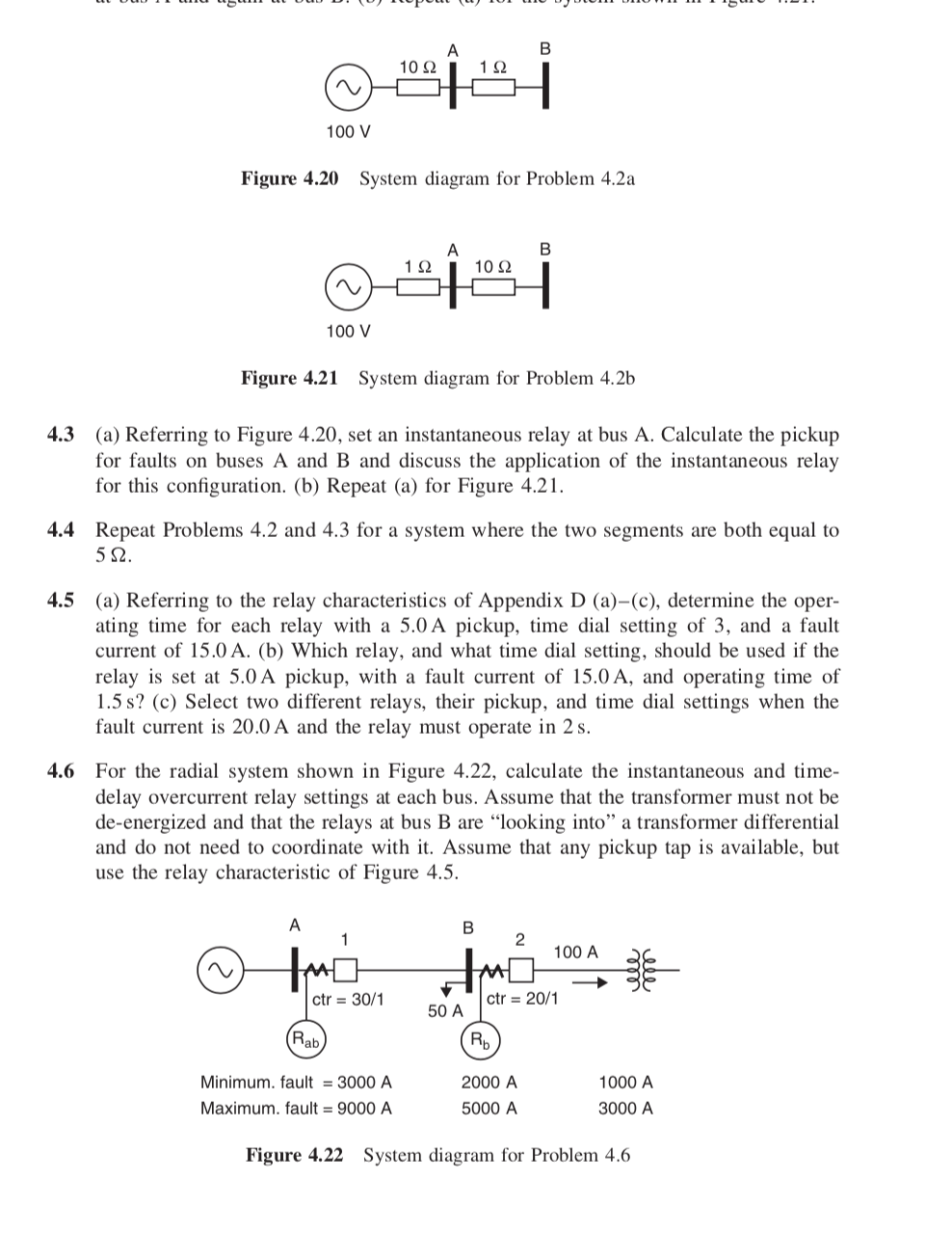# 1012 112 100 V Figure 4.20 System diagram for Problem 4.2a 1 2 10 2 100 V Figure 4.21 System diagram for Problem 4.2b 4.3 (a) Referring to Figure 4.20, set an instantaneous relay at bus A. Calculate the pickup for faults on buses A and B and discuss the application of the instantaneous relay for this configuration. (b) Repeat (a) for Figure 4.21 4.4 Repeat Problems 4.2 and 4.3 for a system where the two segments are both equal to 4.5 (a) Referring to the relay characteristics of Appendix D (a)-(c), determine the oper- ating time for each relay with a 5.0A pickup, time dial setting of 3, and a fault current of 15.0 A. (b) Which relay, and what time dial setting, should be used if the relay is set at 5.0 A pickup, with a fault current of 15.0 A, and operating time of 1.5 s? (c) Select two different relays, their pickup, and time dial settings when the fault current is 20.0 A and the relay must operate in 2s. 4.6 For the radial system shown in Figure 4.22, calculate the instantaneous and time- delay overcurrent relay settings at each bus. Assume that the transformer must not be de-energized and that the relays at bus B are "looking into" a transformer differential and do not need to coordinate with it. Assume that any pickup tap is available, but use the relay characteristic of Figure 4.5. 2 100 A ctr 30/1 50 A ctr-20/1 Minimum.fault 3000 A Maximum. fault 9000 A 2000 A 5000 A 1000 A 3000 A Figure 4.22 System diagram for Problem 4.6

Questionhelp_outlineImage Transcriptionclose1012 112 100 V Figure 4.20 System diagram for Problem 4.2a 1 2 10 2 100 V Figure 4.21 System diagram for Problem 4.2b 4.3 (a) Referring to Figure 4.20, set an instantaneous relay at bus A. Calculate the pickup for faults on buses A and B and discuss the application of the instantaneous relay for this configuration. (b) Repeat (a) for Figure 4.21 4.4 Repeat Problems 4.2 and 4.3 for a system where the two segments are both equal to 4.5 (a) Referring to the relay characteristics of Appendix D (a)-(c), determine the oper- ating time for each relay with a 5.0A pickup, time dial setting of 3, and a fault current of 15.0 A. (b) Which relay, and what time dial setting, should be used if the relay is set at 5.0 A pickup, with a fault current of 15.0 A, and operating time of 1.5 s? (c) Select two different relays, their pickup, and time dial settings when the fault current is 20.0 A and the relay must operate in 2s. 4.6 For the radial system shown in Figure 4.22, calculate the instantaneous and time- delay overcurrent relay settings at each bus. Assume that the transformer must not be de-energized and that the relays at bus B are "looking into" a transformer differential and do not need to coordinate with it. Assume that any pickup tap is available, but use the relay characteristic of Figure 4.5. 2 100 A ctr 30/1 50 A ctr-20/1 Minimum.fault 3000 A Maximum. fault 9000 A 2000 A 5000 A 1000 A 3000 A Figure 4.22 System diagram for Problem 4.6 fullscreen